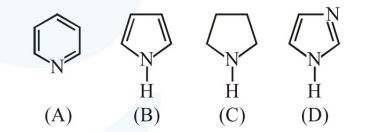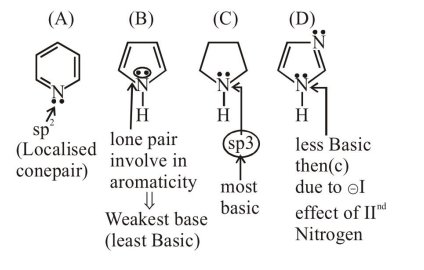# The increasing order of basicity of the following compounds is

Question:

The increasing order of basicity of the following compounds is1. $(\mathrm{A})<(\mathrm{B})<(\mathrm{C})<(\mathrm{D})$

2. $(B)<(A)<(C)<(D)$

3. $(\mathrm{D})<(\mathrm{A})<(\mathrm{B})<(\mathrm{C})$

4. $(\mathrm{B})<(\mathrm{A})<(\mathrm{D})<(\mathrm{C})$

Correct Option: , 4

Solution: